# Winding Factor | Pitch Factor | Distribution Factor

Before knowing about, winding factor, we should know about pitch factor and distribution factor, since winding factor is the product of pitch factor and distribution factor.
If we denote winding factor with Kw, pitch factor with Kp and distribution factor with Kd, we can write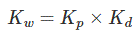The pitch factor and distribution factor are explained below one by one.

## Pitch Factor

In short pitched coil, the induced emf of two coil sides get vectorially added and give resultant emf of the loop. In short pitched coil, the phase angle between the induced emf of two opposite coil sides is less than 180o (electrical). But we know that, in full pitched coil, the phase angle between the induced emf of two coil sides is exactly 180o (electrical).
Hence, the resultant emf of a full pitched coil is just the arithmetic sum of the emfs induced on both sides of the loop. We well know that vector sum or phasor sum of two quantities is always less than their arithmetic sum. The pitch factor is the measure of resultant emf of a short-pitched coil in comparison with resultant emf of a full pitched coil.

Hence, it must be the ratio of phasor sum of induced emfs per coil to the arithmetic sum of induced emfs per coil. Therefore, it must be less than unity.
Let us assume that, a coil is short pitched by an angle α (electrical degree). Emf induced per coil side is E. The arithmetic sum of induced emfs is 2E. That means, 2E, is the induced voltage across the coil terminals, if the coil would have been full pitched.
Now, come to the short pitched coil. From the figure below it is clear that, resultant emf of the short pitched coil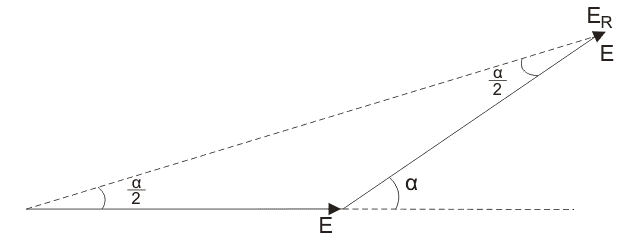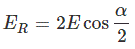Now, as per definition of pitched factor,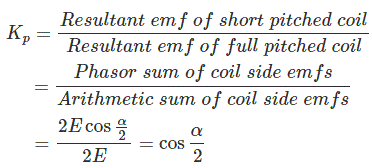This pitch factor is the fundamental component of emf. The flux wave may consist of space field harmonics also, which give rise to the corresponding time harmonics in the generated voltage waveform. A 3rd harmonic component of the flux wave, may be imagined as produced by three poles as compared to one pole for the fundamental component.
In the view of this, the chording angle for the rth harmonic becomes r times the chording angle for the fundamental component and pitch factor for the rth harmonic is given as,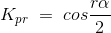The rth harmonic becomes zero, if,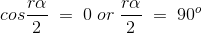In 3 phase alternator, the 3rd harmonic is suppressed by star or delta connection as in the case of 3 phase transformer. Total attention is given for designing a 3 phase alternator winding design, for 5th and 7th harmonics.
For 5th harmonic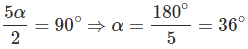For 7th harmonic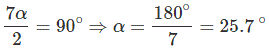Hence, by adopting a suitable chording angle of α = 30o, we make most optimized design armature winding of alternator.

## Distribution Factor

If all the coil sides of any one phase under one pole are bunched in one slot, the winding obtained is known as concentrated winding and the total emf induced is equal to the arithmetic sum of the emfs induced in all the coils of one phase under one pole.
But in practical cases, for obtaining smooth sinusoidal voltage waveform, armature winding of alternator is not concentrated but distributed among the different slots to form polar groups under each pole. In distributed winding, coil sides per phase are displaced from each other by an angle equal to the angular displacement of the adjacent slots. Hence, the induced emf per coil side is not an angle equal to the angular displacement of the slots.
So, the resultant emf of the winding is the phasor sum of the induced emf per coil side. As it is phasor sum, must be less than the arithmetic sum of these induced emfs.
Resultant emf would be an arithmetic sum if the winding would have been a concentrated one.
As per definition, distribution factor is a measure of resultant emf of a distributed winding in compared to a concentrated winding.

We express it as the ratio of the phasor sum of the emfs induced in all the coils distributed in some slots under one pole to the arithmetic sum of the emfs induced. Distribution factor is,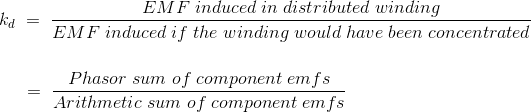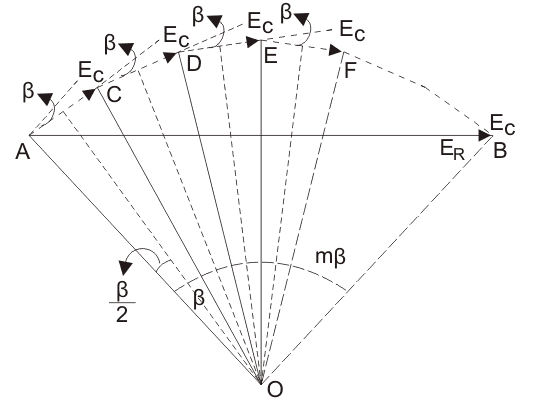As pitch factor, distribution factor is also always less than unity.
Let the number of slots per pole is n.
The number of slots per pole per phase is m.
Induced emf per coil side is Ec.
Angular displacement between the slots,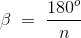Let us represent the emfs induced in different coils of one phase under one pole as AC, DC, DE, EF and so on. They are equal in magnitude, but they differ from each other by an angle β.
If we draw bisectors on AC, CD, DE, EF ——–. They would meet at common point O.
Emf induced in each coil side,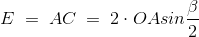As the slot per pole per phase is m, the total arithmetic sum of all induced emfs per coil sides per pole per phase,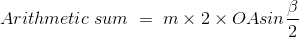The resultant emf would be AB, as represented by the figure,
Hence, the resultant emf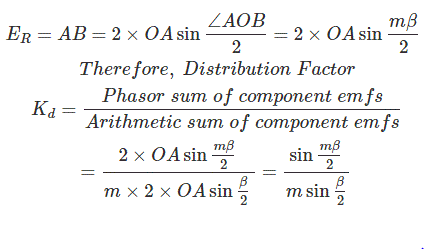mβ is also known as the phase spread in electrical degree.
The distribution factor Kd given by equation is for the fundamental component of emf.
If the flux distribution contains space harmonics the slot angular pitch β on the fundamental scale, would become rβ for the rth harmonic component and thus the distribution factor for the rth harmonic would be.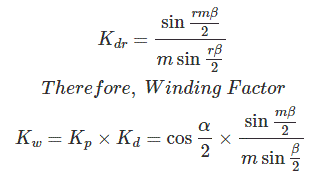Want To Learn Faster? 🎓
Get electrical articles delivered to your inbox every week.
No credit card required—it’s 100% free.